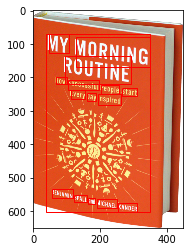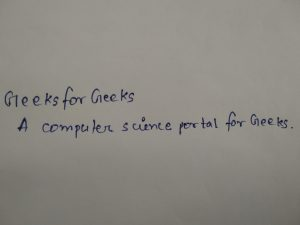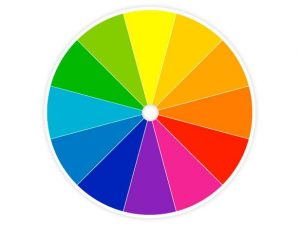GeeksforGeeks App
Open AppBrowser
Continue

# How to use Vision API from Google Cloud | Set-2

In the previous article we have seen how to use Facial Detection, Logo Detection, Label Detection and Landmark Detection features of Vision using Vision API, now let’s see few more features like Optical Character Recognition, handwritten text detection, Image Properties Detection etc.

### Text Detection (Optical Character Recognition):

It detects and extracts text from within an image.

 `import` `os``import` `io``from` `google.cloud ``import` `vision``from` `matplotlib ``import` `pyplot as plt``from` `matplotlib ``import` `patches as pch`` ` `os.environ[``'GOOGLE_APPLICATION_CREDENTIALS'``] ``=` `     ``os.path.join(os.curdir, ``'credentials.json'``)`` ` `client ``=` `vision.ImageAnnotatorClient()`` ` `f ``=` `'image_filename.jpg'``with io.``open``(f, ``'rb'``) as image:``    ``content ``=` `image.read()``     ` `image ``=` `vision.types.Image(content ``=` `content)``response ``=` `client.text_detection(image ``=` `image)``texts ``=` `response.text_annotations`` ` `a ``=` `plt.imread(f)``fig, ax ``=` `plt.subplots(``1``)``ax.imshow(a)`` ` `for` `text ``in` `texts:``    ``print``(text.description)``     ` `    ``vertices ``=` `([(vertex.x, vertex.y) ``                 ``for` `vertex ``in` `text.bounding_poly.vertices]) ``   ` `    ``print``(``'Vertices covering text: {}\n\n'``.``format``(vertices))``    ``rect ``=` `pch.Rectangle(vertices[``0``], (vertices[``1``][``0``] ``-` `vertices[``0``][``0``]), ``                        ``(vertices[``2``][``1``] ``-` `vertices[``0``][``1``]), linewidth ``=` `1``, ``                                       ``edgecolor ``=``'r'``, facecolor ``=``'none'``) ``    ``ax.add_patch(rect)``     ` `plt.show()`

The above code extracts text from a given image and also prints the coordinates of the vertices of the rectangle containing the text.
For example, when the following image is given as input:Output:

```MY MORNING
ROUTINE
How Successful People Start
Every Day Inspired
BENJAMIN SPALL and MICHAEL XANDER

Vertices covering text: [(38, 71), (348, 71), (348, 602), (38, 602)]

MY
Vertices covering text: [(46, 71), (108, 82), (100, 128), (38, 117)]

MORNING
Vertices covering text: [(129, 79), (348, 118), (338, 170), (120, 131)]

ROUTINE
Vertices covering text: [(96, 135), (292, 170), (283, 219), (87, 184)]

How
Vertices covering text: [(68, 200), (101, 205), (98, 221), (65, 216)]

Successful
Vertices covering text: [(104, 207), (196, 222), (193, 238), (101, 224)]

People
Vertices covering text: [(202, 222), (257, 231), (254, 251), (199, 242)]

Start
Vertices covering text: [(265, 232), (311, 239), (309, 255), (262, 248)]

Every
Vertices covering text: [(112, 238), (155, 246), (152, 265), (109, 258)]

Day
Vertices covering text: [(160, 246), (189, 251), (185, 271), (157, 266)]

Inspired
Vertices covering text: [(194, 251), (262, 263), (258, 283), (191, 271)]

BENJAMIN
Vertices covering text: [(57, 534), (118, 546), (115, 561), (54, 549)]

SPALL
Vertices covering text: [(122, 550), (160, 558), (157, 572), (119, 564)]

and
Vertices covering text: [(165, 560), (185, 564), (182, 577), (162, 573)]

MICHAEL
Vertices covering text: [(190, 564), (250, 576), (247, 590), (187, 578)]

XANDER
Vertices covering text: [(254, 575), (311, 587), (308, 602), (251, 591)]```### Document/handwritten text detection:

This feature also performs Optical Character Recognition on dense documents, including handwritings.

 `import` `os``import` `io``from` `google.cloud ``import` `vision``from` `matplotlib ``import` `pyplot as plt`` ` `os.environ[``'GOOGLE_APPLICATION_CREDENTIALS'``] ``=` `     ``os.path.join(os.curdir, ``'credentials.json'``)`` ` `client ``=` `vision.ImageAnnotatorClient()`` ` `f ``=` `'image_filename.jpg'``with io.``open``(f, ``'rb'``) as image:``    ``content ``=` `image.read()``     ` `image ``=` `vision.types.Image(content ``=` `content)``response ``=` `client.document_text_detection(image ``=` `image)`` ` `a ``=` `plt.imread(f)``plt.imshow(a)`` ` `txt ``=` `[]``for` `page ``in` `response.full_text_annotation.pages:``        ``for` `block ``in` `page.blocks:``            ``print``(``'\nConfidence: {}%\n'``.``format``(block.confidence ``*` `100``))``            ``for` `paragraph ``in` `block.paragraphs:`` ` `                ``for` `word ``in` `paragraph.words:``                    ``word_text ``=` `''.join([symbol.text ``for` `symbol ``in` `word.symbols])``                    ``txt.append(word_text)``                     ` `print``(txt)`

The above code identifies and extracts handwritten texts from an image and outputs it.
For example, when we give the following image as input:Output:

```Block confidence: 97.00000286102295%

['Geeks', 'for', 'Geeks', 'A', 'computer', 'science', 'portal', 'for', 'Geeks', '.']```

### Image Properties Detection:

This feature detects the general attributes of an image, like dominant color.

 `import` `os``import` `io``from` `google.cloud ``import` `vision``from` `matplotlib ``import` `pyplot as plt`` ` `os.environ[``'GOOGLE_APPLICATION_CREDENTIALS'``] ``=``      ``os.path.join(os.curdir, ``'credentials.json'``)`` ` `client ``=` `vision.ImageAnnotatorClient()`` ` `f ``=` `'image_filename.jpeg'``with io.``open``(f, ``'rb'``) as image:``    ``content ``=` `image.read()``     ` `image ``=` `vision.types.Image(content ``=` `content)`` ` `response ``=` `client.image_properties(image ``=` `image)``properties ``=` `response.image_properties_annotation`` ` `a ``=` `plt.imread(f)``plt.imshow(a)`` ` `for` `color ``in` `properties.dominant_colors.colors:``        ``print``(``'fraction: {}'``.``format``(color.pixel_fraction))``        ``print``(``'\tr: {}'``.``format``(color.color.red))``        ``print``(``'\tg: {}'``.``format``(color.color.green))``        ``print``(``'\tb: {}'``.``format``(color.color.blue))`

The code takes an image as in input and returns its color properties i.e. amount of red, green and blue colors. For example, when the following image is given as input:Output:

```fraction: 0.036332178860902786
r: 5.0
g: 185.0
b: 6.0
fraction: 0.03337658569216728
r: 131.0
g: 207.0
b: 13.0
fraction: 0.029988465830683708
r: 253.0
g: 169.0
b: 5.0
fraction: 0.0262399073690176
r: 254.0
g: 123.0
b: 5.0
fraction: 0.03553921729326248
r: 253.0
g: 248.0
b: 12.0
fraction: 0.02104959636926651
r: 249.0
g: 36.0
b: 6.0
fraction: 0.024581892415881157
r: 3.0
g: 35.0
b: 188.0
fraction: 0.03424163907766342
r: 6.0
g: 122.0
b: 200.0
fraction: 0.027032872661948204
r: 140.0
g: 32.0
b: 185.0
fraction: 0.029411764815449715
r: 10.0
g: 177.0
b: 217.0```

### Safe Search Properties Detection:

Detects explicit content such as adult content or violent content within an image. This feature uses five categories (“adult”, “spoof”, “medical”, “violence”, and “racy”) and returns the likelihood that each is present in a given image.

 `import` `os``import` `io``from` `google.cloud ``import` `vision``from` `matplotlib ``import` `pyplot as plt`` ` `os.environ[``'GOOGLE_APPLICATION_CREDENTIALS'``] ``=` `    ``os.path.join(os.curdir, ``'credentials.json'``)`` ` `client ``=` `vision.ImageAnnotatorClient()`` ` `f ``=` `'image_filename.jpg'``with io.``open``(f, ``'rb'``) as image:``    ``content ``=` `image.read()``     ` `image ``=` `vision.types.Image(content ``=` `content)`` ` `a ``=` `plt.imread(f)``plt.imshow(a)`` ` `response ``=` `client.safe_search_detection(image ``=` `image)``safe ``=` `response.safe_search_annotation`` ` `likelihood_name ``=` `(``'UNKNOWN'``, ``'VERY_UNLIKELY'``, ``'UNLIKELY'``,``                   ``'POSSIBLE'``, ``'LIKELY'``, ``'VERY_LIKELY'``)`` ` `print``(``'Adult: {}'``.``format``(likelihood_name[safe.adult]))``print``(``'Medical: {}'``.``format``(likelihood_name[safe.medical]))``print``(``'Spoofed: {}'``.``format``(likelihood_name[safe.spoof]))``print``(``'Violence: {}'``.``format``(likelihood_name[safe.violence]))``print``(``'Racy: {}'``.``format``(likelihood_name[safe.racy]))`

Given an image, the code will determine the probability of it being an image with graphics or adult content.

### Object Detection:

Detects and extracts multiple objects from an image. It localizes multiple objects and returns their coordinates.

 `import` `os``import` `io``from` `google.cloud ``import` `vision``from` `matplotlib ``import` `pyplot as plt`` ` `os.environ[``'GOOGLE_APPLICATION_CREDENTIALS'``] ``=` `      ``os.path.join(os.curdir, ``'credentials.json'``)`` ` `client ``=` `vision.ImageAnnotatorClient()`` ` `f ``=` `'image_filename.jpg'``with io.``open``(f, ``'rb'``) as image:``    ``content ``=` `image.read()``     ` `image ``=` `vision.types.Image(content ``=` `content)`` ` `a ``=` `plt.imread(f)``plt.imshow(a)`` ` `response ``=` `client.object_localization(image ``=` `image)``objects ``=` `response.localized_object_annotations`` ` `print``(``'Number of objects found: '``, ``len``(objects))``for` `object_ ``in` `objects:``    ``print``(``'Object: '``, object_.name)``    ``print``(``'Confidence: '``, object_.score)`

For example, when we input the following image:Output:

```Number of objects found:  1
Object:  Scissors
Confidence:  0.540185272693634```